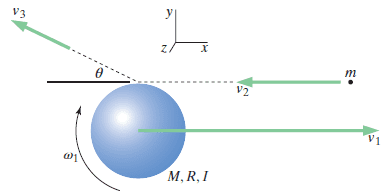# Finding angular velocity after a collision

## Homework Statement

[/B]
A spherical satellite of approximately uniform density with radius 5.1 m and mass 280 kg is originally moving with velocity2600, 0, 0m/s, and is originally rotating with an angular speed 2 rad/s, in the direction shown in the diagram. A small piece of space junk of mass 7.6 kg is initially moving toward the satellite with velocity−2200, 0, 0m/s. The space junk hits the edge of the satellite as shown in the figure below, and moves off with a new velocity−1300, 480, 0m/s. Both before and after the collision, the rotation of the space junk is negligible.(a) Just after the collision, what are the components of the center-of-mass velocity of the satellite (vx and vy) and its rotational speed ω? (For vx, enter your answer to at least four significant figures.)
(b) Calculate the rise in the internal energy of the satellite and space junk combined.

## Homework Equations

From Wiley Matter and Interactions, 4e, vol 1, p. 434-35:
## P_{xf} = P_{xi} ##
## v_{s,xf} = v_{s,xi} + \frac{m}{M}(v_{j,xi} - v_{j,xf}) cos \theta ##

## P_{yf} = P_{yi} ##
## v_{s,yf} = v_{s,yi} + \frac{m}{M}(v_{j,yi} - v_{j,yf}) sin \theta ##

No net torques:
## L_f = L_i ##
## I \omega_i + hmv_1 sin(90 - \theta) = I \omega_f + hmv_2 sin(90 - \theta) ##
Solid sphere: ## I = \frac{2}{5}MR^2 ##
## \omega_f = \omega_i + \frac{hm}{\frac{2}{5}MR^2}(v_1 - v_2) cos \theta ##

## The Attempt at a Solution

I have already viewed the key and have the answers, but I still have questions on how to find the answer.
I was able to get Vx and Vy for the satelite using the above equations. I used theta=0, and used cos theta for the y component. I'm not sure why this worked though.
## v_{s,xf} = 2600 + \frac{7.6}{280}(-2200 + 1300) cos 0 = 2575.57 m/s ##
## v_{s,yf} = 0 + \frac{7.6}{280}(0 - 480) cos 0 = -13.03 m/s ##
These are both correct according to the key.

I did not get the correct answer for the change in angular velocity. I used
## \omega_f = 2 + \frac{(5.1)(7.6)}{2913.12}(2200 - 1385.78) cos 0 = 12.83 rad/s, +\hat{z} ##
The correct answer according to the key is 9.97 rad/s, no direction given.

I could find the difference in energy after I had the correct angular velocity of the satellite. There are no external forces so no work is being done, so the only difference in energy is the difference in kinetic energy. I calculated this using the given correct angular velocity of the satellite and got the right answer, 2.86e+7.

Last edited by a moderator:

TSny
Homework Helper
Gold Member

## Homework Equations

From Wiley Matter and Interactions, 4e, vol 1, p. 434-35:
## P_{xf} = P_{xi} ##
## v_{s,xf} = v_{s,xi} + \frac{m}{M}(v_{j,xi} - v_{j,xf}) cos \theta ##

## P_{yf} = P_{yi} ##
## v_{s,yf} = v_{s,yi} + \frac{m}{M}(v_{j,yi} - v_{j,yf}) sin \theta ##
The above equations are not correct. Start from ## P_{xf} = P_{xi} ## and derive the correct equation that should take the place of ## v_{s,xf} = v_{s,xi} + \frac{m}{M}(v_{j,xi} - v_{j,xf}) cos \theta ##.

Likewise for ## P_{yf} = P_{yi} ##.

## L_f = L_i ##
## I \omega_i + hmv_1 sin(90 - \theta) = I \omega_f + hmv_2 sin(90 - \theta) ##

Again, this isn't correct if the ##\theta## on the left side is the same angle as the ##\theta## on the right side. Try setting up your own equation for ## L_f = L_i ##.